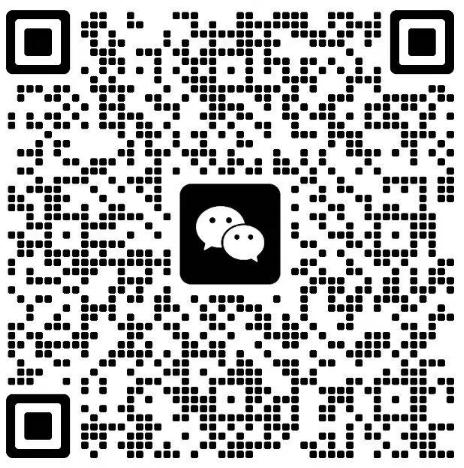# 2022年浙江成人高考高中起点理科《数学》试题及答案②6.下列函数中，为偶函数的是()

A y=3x-1

B.y=x-3

c.y=3

D.y=log;x

B.C.D项均为非奇非偶函数，只有A项为偶函数。

7.二次函数y=-2(x-3)+1的图像是由函数y=-2x的图像经过下列哪项平移得到的()

A.先向右平移3个单位，再向上平移1个单位

B.先向左平移3个单位，再向上平移1个单位

c.先向右平移3个单位，再向下平移1个单位

D.先向左平移3个单位，再向下平移1个单位

y=-2x向右平移3个单位得到y=-2(x-3)2,

y=-2(x-3)再向上平移1个单位得到y=-2(x-3)2+1.

8.设等比数列{a,}的公比q=2,且a:*a=8,则=()

A.8

B.16

c.32

D.64

9.已知点4(2,2),B(-5,9),则线段AB的垂直平分线的方程是()

A.x-y+7=0

B.x+y-7=0

c.2x-y+7=0

D.x+2y+7=0

9-2=-1,//，又因为k=线段AB的中点坐标是(一-5-2)故AB的垂直平分线的的斜率为1,由直线的斜截式可知其方程为11，=即x-y+7=0.+xC-

10.圆x+y2+2x-8y+8=0的半径为()

A.1

B.3

C.4

D.6

x+y2+2x-8y+8=0=>x+2x+1+y-8y+16=9=(x+1)+(y-4)=33,故圆的半径为3.

2023年全国成人高考还有

### 浙江成考网在线客服